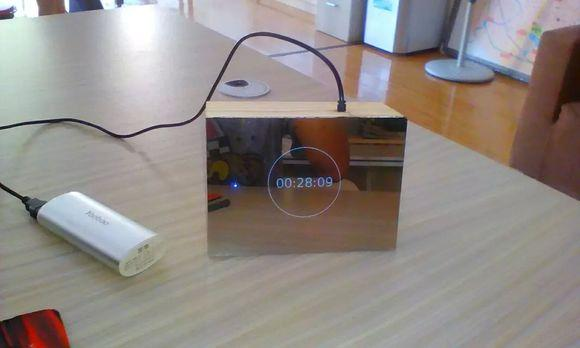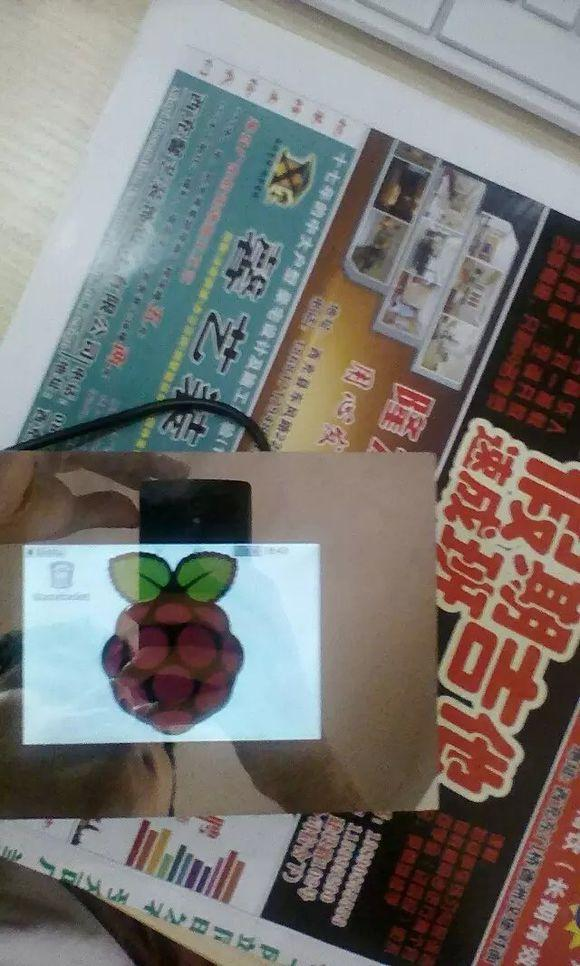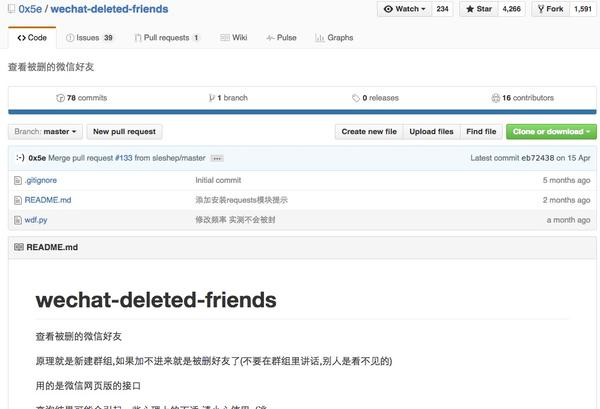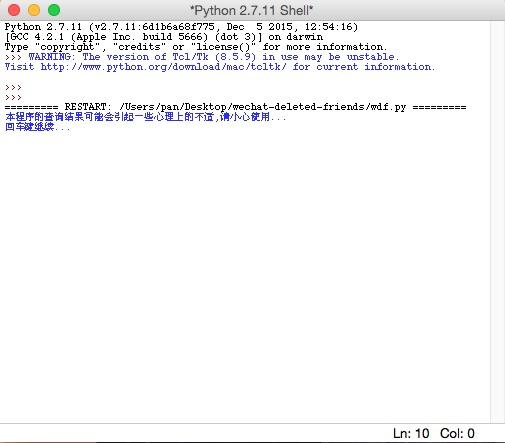# 用python写过哪些脑洞大开的小工具？为什么知乎不支持markdown语言—破百了就更新—这货叫做 树莓派镜子他其实是由原子镜+lcd屏幕+树莓派+木头盒子 组成的我们先来说原子镜python给我很大的惊喜

python写了个命令行麻将cugb1004101218/majiang · github半成品，不能碰，屁胡。#!/usr/bin/env python2.7
# –*– encoding: utf-8 –*–
# enable print(,end=”)
#from __future__ import print_function
import argparse
import sys
import os
import re
import bs4
import urlparse
import requests
#out = url.split(‘/’)[-1]
resp = requests.get(url, stream=true)
if resp.status_code != requests.codes.ok:
return false
if total is none:
total = 0
else:
total = int(total)
def show_progress(total, acc):
if total == 0:
sys.stdout.write(“{} ?\r”.format(out))
else:
#sys.stdout.write(“{} [ {:3d}% ]\r”.format(100*acc/total, out))
sys.stdout.write(“{} \”{}\” {:3d}%\r”.format(title, out, 100*acc/total))
sys.stdout.flush()
acc = 0
show_progress(total, acc)
with open(out, ‘wb’) as f:
for chunk in resp.iter_content(chunk_size=1024):
if chunk: # filter out keep-alive new chunks
f.write(chunk)
f.flush()
acc += len(chunk)
show_progress(total, acc)
sys.stdout.write(“\n”)
return true
def fetch(from_url, offset, count):
with open(from_url, “r”) as f:
soup = bs4.beautifulsoup(html)
# get title
content = soup.find_all(“h2″, class_=”first”)
if len(content) == 0:
return
elif len(content) != 1:
print(“title not unique!”)
fdir = content.contents.encode(“utf8”).strip().replace(“–”, “-“)
if not os.path.exists(fdir):
os.mkdir(fdir)
print(“mkdir: \”{}\””.format(fdir))
# get image url
content = soup.find_all(“p”, ^post-“))
if len(content) == 0:
return
elif len(content) != 1:
print(“target not unique!”)
imgs = content.find_all(“img”)
start = 0 if offset is none else offset
end = len(imgs) if count is none else (start+count)
print(“# of image: {}”.format(nimg))
for idx in range(start, end):
img = imgs[idx]
url = str(img.attrs[“src”])
#url = url.replace(“http://t10”, “http://i10”)
url = url.replace(“http://t”, “http://i”)
ext = url[url.rfind(“.”):]
out = “{0}/{1}{2}”.format(fdir, idx+1, ext)
# get image file
for retry in range(0, 5):
title = (“({{:{}d}}/{{}})”.format(len(str(end))).format(idx+1, end))
break
print(“failed, try again ({})”.format(retry+1))
def main():
parser = argparse.argumentparser(description=’nah’)
args = parser.parse_args()
for url in args.urls:
fetch(url, args.offset, args.count)
if __name__ == “__main__”:
main()

import image
color = ‘mnhq\$oc?7>!:-;.’ #zifu
def to_html(func):

”’
html_tail = ”
# ding yi html
def wrapper(img):
pic_str = func(img)
pic_str = ”.join(l + ‘ ‘ for l in pic_str.splitlines())
return html_head + pic_str + html_tail
return wrapper
# fan hui zhi
@to_html
def make_char_img(img):
pic_str = ”
width, height = img.size
for h in xrange(height):
for w in xrange(width):
pic_str += color[int(pix[w, h]) * 14 / 255]
pic_str += ‘\n’
return pic_str
def preprocess(img_name):
img = image.open(img_name)
w, h = img.size
m = max(img.size)
delta = m / 200.0
w, h = int(w / delta), int(h / delta)
img = img.resize((w, h))
img = img.convert(‘l’)
return img
def save_to_file(filename, pic_str):
outfile = open(filename, ‘w’)
outfile.write(pic_str)
outfile.close()
def main():